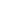Get Flat 50% OFF

Ends in

•• 11th
•• Mathematics
• Back to Doubt Clearing

Q1$log_5 {\sqrt {5 \sqrt {5} \sqrt {5....∞}}} = ?$Q2$log_x (2x^2-9)=2$  find $x$ ?Q3  $_{10}log_{100}^{16} = ?$

Asked By Alamanchili Yudhi Vishnu Bhargav

Updated Thu, 13 Dec 2018 02:36 pm